﻿ 十二、指針和引用_ZenDei技術網路在線

# 十二、指針和引用#### 十二、指針和引用（二）

##### 1、指針和數組的關係

1）思考

​ 假設你要設計一種編程語言，你要如何實現數組呢？思考之前請先牢記：數組在記憶體中是連續的，維度由低到高（大部分操作系統下）。

2）彙編分析數組如何實現

``````//C++代碼
#include <iostream>

int main()
{
int a{};
int* ptrA{ &a };
*ptrA = 5;    //通過指針設置數組的值

a = 5;     //通過數組下標設置數組的值
a = 5;
}

//上述代碼彙編分析
int a{};
int* ptrA{ &a };
00A51840  mov         eax,4                     //eax=4
00A51845  imul        ecx,eax,0                 //imul為乘法,即ecx=eax*0=0
00A51848  lea         edx,[ebp+ecx-1Ch]         //edx=ebp+ecx-1Ch=ebp-1Ch
00A5184C  mov         dword ptr [ebp-28h],edx   //ptr表示指針，即[ebp-28h]=ebp-1Ch, []中的表示地址
*ptrA = 5;
00A5184F  mov         eax,dword ptr [ebp-28h]    //eax=[ebp-28h] 即eax=ebp-1Ch
00A51852  mov         dword ptr [eax],5         //[eax]=5,即[ebp-1Ch]=5，即a=5

a = 5;
00A51858  mov         eax,4                    //eax=4
00A5185D  imul        ecx,eax,0                //ecx=eax*0=0
00A51860  mov         dword ptr [ebp+eax-1Ch],5    //[ebp+eax-1Ch]=5,即[ebp-1Ch]=5
a = 5;
00A51868  mov         eax,4                    //eax=4
00A5186D  shl         eax,0                    //shl為左位移，即eax<<0,即eax=eax*2^0即eax=eax*1=4
00A51870  mov         dword ptr a[ebp+eax-1Ch],5   //[a]=5
}
``````

``````//數組a的地址本質上和a的地址相等。
#include <iostream>

int main()
{
int a{};
int* ptrA{ &a };
*ptrA = 5;    //通過指針設置數組的值

std::cout << "數組a的地址為：" << a << std::endl;
std::cout << "a的地址為： " << &a << std::endl;
}
//註：因數組a的地址和a的地址一樣，所以ptrA和a在本質上一樣，所以在定義指針數組時，可以直接寫為int* ptrA {a}; ptrA=1002;

``````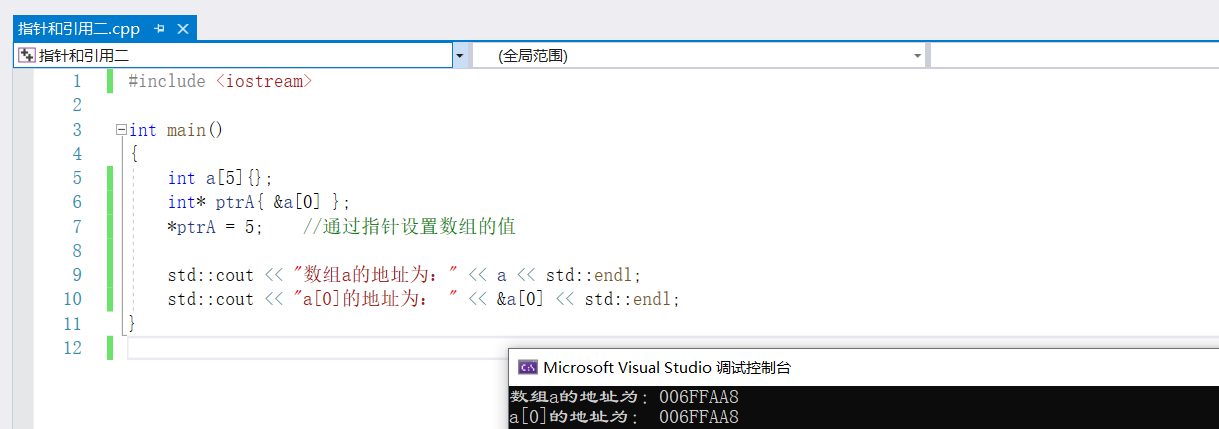3）結論：指針可以當數組用

​ 數組的底層實現是利用了指針，因此，我們甚至可以大膽的說，起始C/C++里根本不存在什麼數組，所謂的數組不過是利用指針玩的小把戲而已。

​ 從原理上來講，指針和數組是同一個方法的不同表達，而數組名本身就是一個指針，數組元素只是這個指針按照一定的偏移量後，對應的記憶體區域里的內容。

​ 因此我們嘗試一下按照數組的使用方式來使用一下指針，看看會發生什麼事情。

``````#include <iostream>
int main()
{
int a{};
int* ptrA{ a };
*ptrA = 5;
a = 5;
a = 50001;
a = 5;

std::cout << a << std::endl;     //010FF9F8
std::cout << &a << std::endl;  //010ff9F8
std::cout << ptrA << std::endl; //50001
std::cout << a << std::endl;  //50001

std::cout << sizeof(a) << std::endl;     //20
std::cout << sizeof(ptrA) << std::endl;  //4

}
``````

4）多維數組思考

​ 數組的本質是連續的記憶體區域，所以我們可以大膽設想，所謂的多維數組起始不存在，多維只是我們人類為了方便我們自己的理解創造出來的邏輯方法。

!

5）數組在記憶體中的表現形式

​ 不管是int a還是int a在記憶體中的本質都是一樣的，都是一塊連續的記憶體空間，並且占用的地址也都一樣。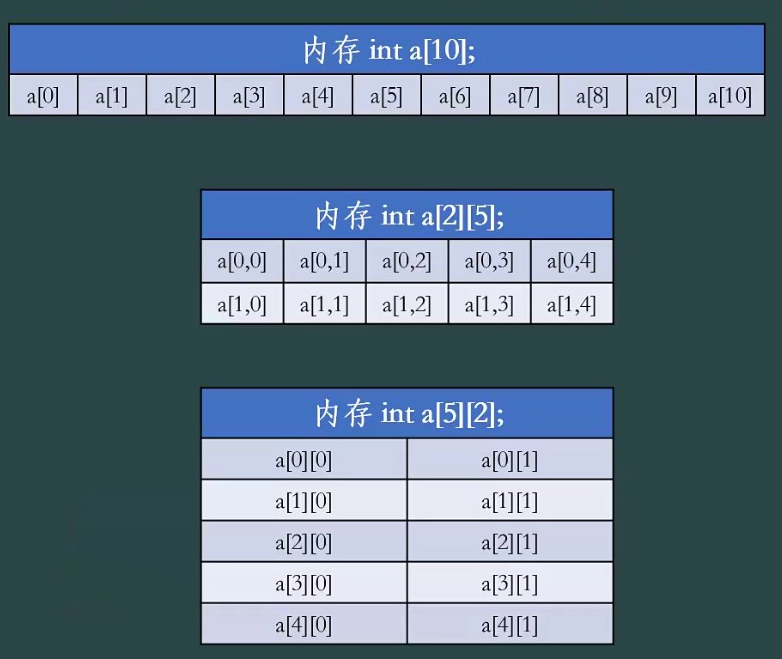``````#include <iostream>

int main()
{
int test
{
{1001,1002,1003,1004,1005},
{2001,2002,2003,2004,2005}    //多維數組在記憶體中的人為邏輯排序
};
//1001, 1002, 1003, 1004, 1005,2001,2002,2003,2004,2005   記憶體中多維數組的真實排序

int* ptrTest{ (int*)test };  //可以進行強制類型轉化，因為test本質是一個地址，裡面保存中test的地址
//輸入test數組最後一個元素
std::cout << "通過數組下標獲取test最後一個元素：" << test << std::endl;
std::cout << "通過指針獲取test最後一個元素：    " << ptrTest << std::endl;
}

//test是一個數組指針
``````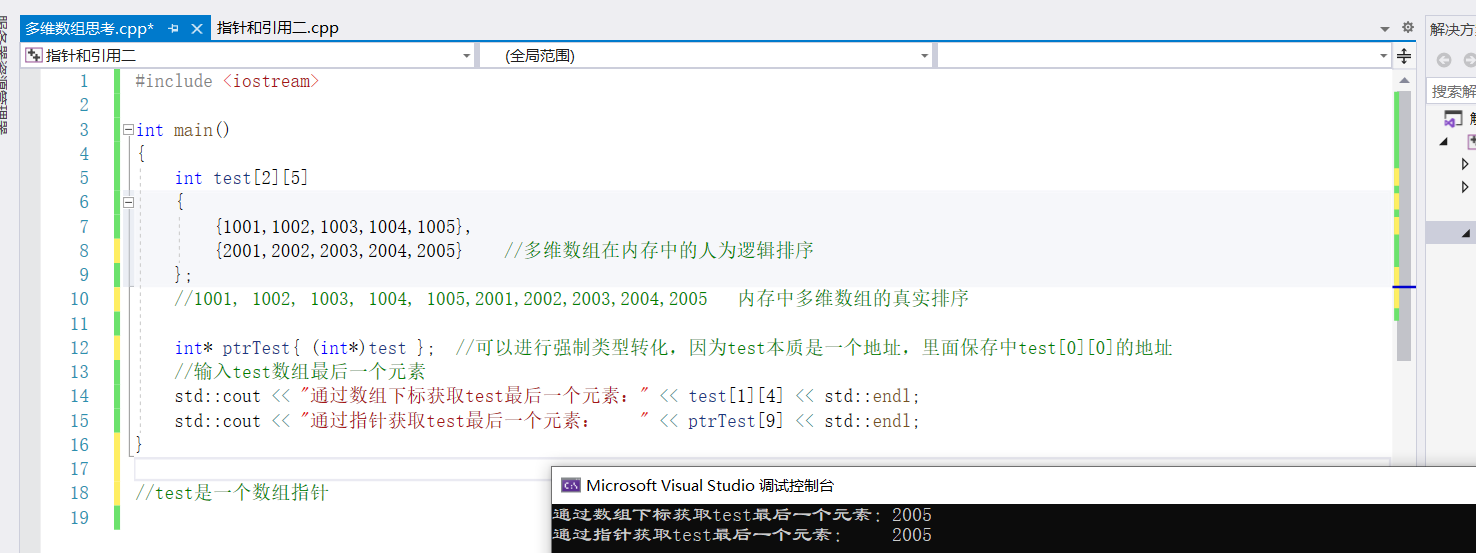6）數組指針和指針數組

①指針數組：指針數組本質上來講是一個數組，數組的內容是指針，如int* ptrA;

②數組指針：數組指針本質上來講是一個指針，只是這個指針用來處理數組問題

``````//數組指針定義
int (*ptrB);
``````
``````//數組指針使用
#include <iostream>
int main()
{
int test
{
{1001,1002,1003,1004,1005},
{2001,2002,2003,2004,2005}
};

int* ptestA;  //指針數組，本質上是一個數組，即將數組中存放5個地址

int(*pTestA){ test }; //數組指針(本質上是一個指針)，表示每一行按照5個元素斷開，即該指針處理每一行5個數據的數組
std::cout << test << std::endl;        //2005
std::cout << pTestA << std::endl;      //1002
std::cout << sizeof(pTestA) << std::endl;      //4, 數組指針是一個指針，指針大小為4

std::cout << pTestA << std::endl;
pTestA = pTestA + 1;               //指針加1，表示加對應數組類型的大小，即+1*數據類型大小*數組指針元素個數，即1*4*5=20
std::cout << pTestA << std::endl;
}
``````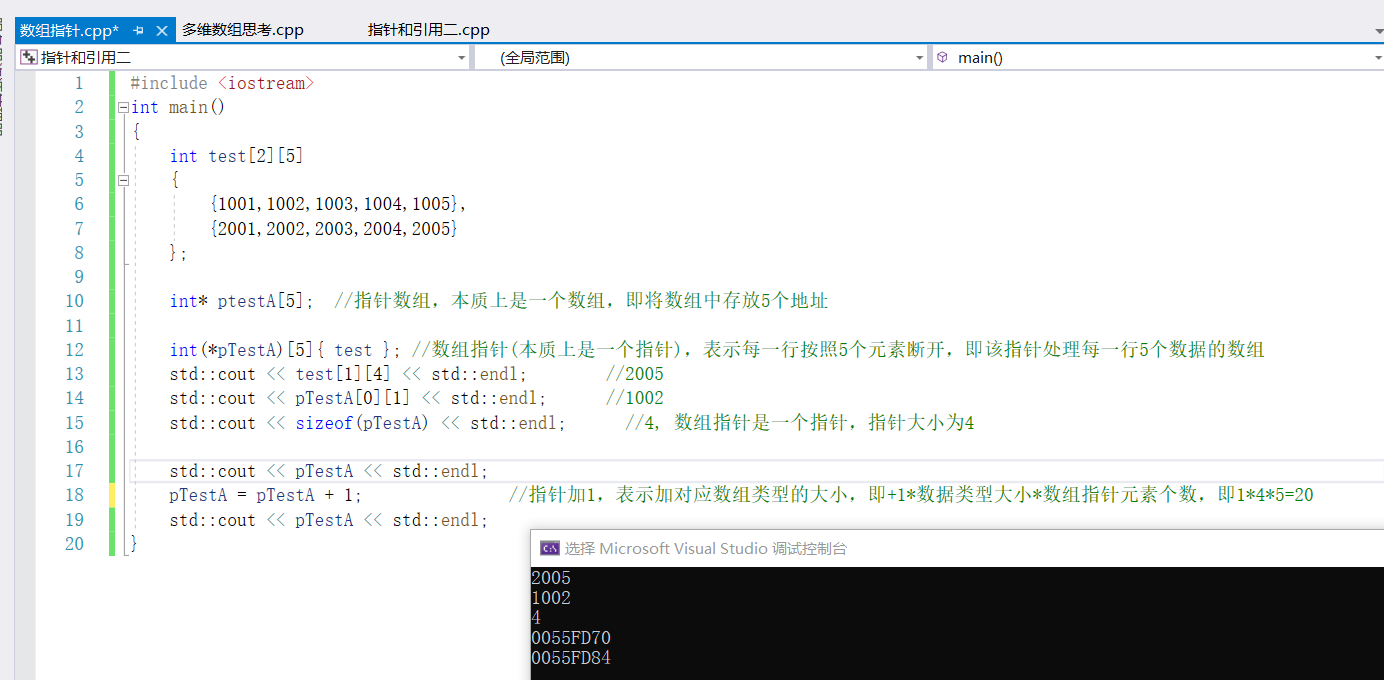##### 2、動態記憶體分配

1）項目設計

​ 麟江湖副本系統 ：玩家進入麟江湖副本系統後，地圖上會隨機刷新一批怪物，數量不等，從100-10000個都有可能，我們如何應對這種不確定數量對象的問題？

2）C語言中的動態記憶體分配malloc

``````//C語言中的動態記憶體分配malloc語法
void* malloc(size_t size);  //malloc將為用戶分為size_t位元組個記憶體，並且返回記憶體分配的地址，如果分配失敗，那麼返回0

//示例
int* pa=(int*)malloc(4);  //pa是分配好的記憶體的地址，4是要分配的記憶體的大小，如果記憶體分配失敗，那麼pa=0

typedef unsigned int     size_t;
``````

malloc的簡單使用：

``````//malloc的簡單使用
#include <iostream>
int main()
{
unsigned x;
std::cout << "請輸入要申請的記憶體大小：";
std::cin >> x;
//malloc(x*4); //放什麼類型的變數，就*什麼類型的變數大小，如int類型*4。可直接使用seizeof(int)計算
int* p = (int*)malloc(sizeof(int) * x);   //void*  是空類型的指針,即沒有任何類型，無法將其賦值給int*類型的指針，因此需要強制類型轉化

//記憶體不一定分配成功
if (p == nullptr)  //nullptr就相當於0，但是推薦使用nullptr，C語言中只能使用0
{
std::cout << "記憶體分配失敗！！！" << std::endl;
}
else      //記憶體分配成功後，再執行相關操作
{
p = 952;
p = 953;
p = p * p;
std::cout<<"p:" << p << " p:" << p << " p:" << p << std::endl;
}

}
``````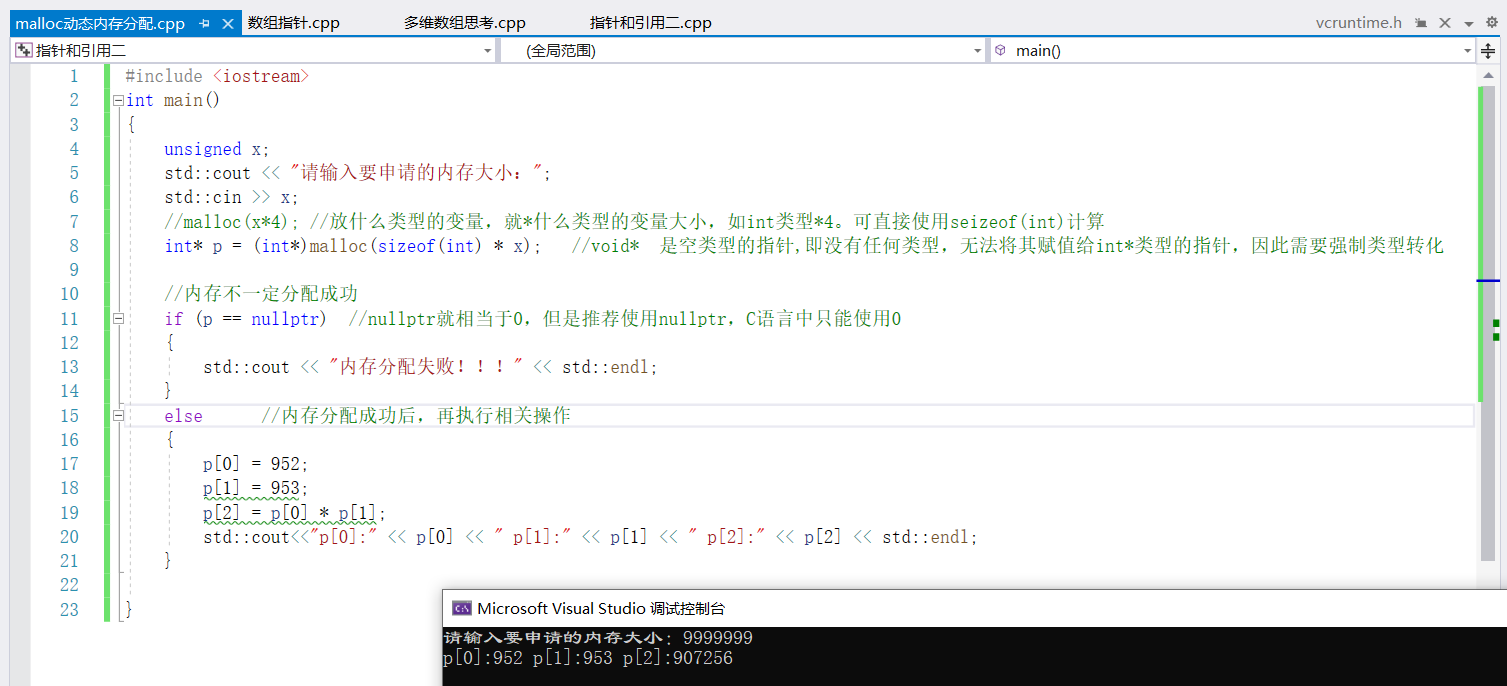3）C語言中的動態記憶體分配callloc

``````//動態記憶體分配函數callloc語法
void* calloc(size_t count,size_t size);  //calloc將為用戶分配count乘size_t位元組個記憶體，並且返回記憶體分配的地址，如果分配失敗，那麼返回0

//calloc示例
int* pa=(int*)calloc(1,4); //pa是分配好的記憶體地址，1是要分配的元素個數，4是要分配的每個元素個數的大小

``````
``````//動態記憶體分配callloc示例
#include <iostream>
int main()
{
unsigned x;
std::cout << "請輸入要申請的記憶體大小：";
std::cin >> x;
//calloc直接說明需要申請的記憶體個數，及每個記憶體的大小
int* p = (int*)calloc(x, sizeof(int));
//calloc會將自動分配的記憶體空間的值設置為：0
std::cout << "calloc會將自動分配的記憶體空間的值設置為：" << p << std::endl;
if (p == nullptr)
{
std::cout << "記憶體分配失敗！！！";
}
else
{
p = 952;
p = 953;
p = p * p;
std::cout << "p:" << p << " p:" << p << " p:" << p << std::endl;
}
}
``````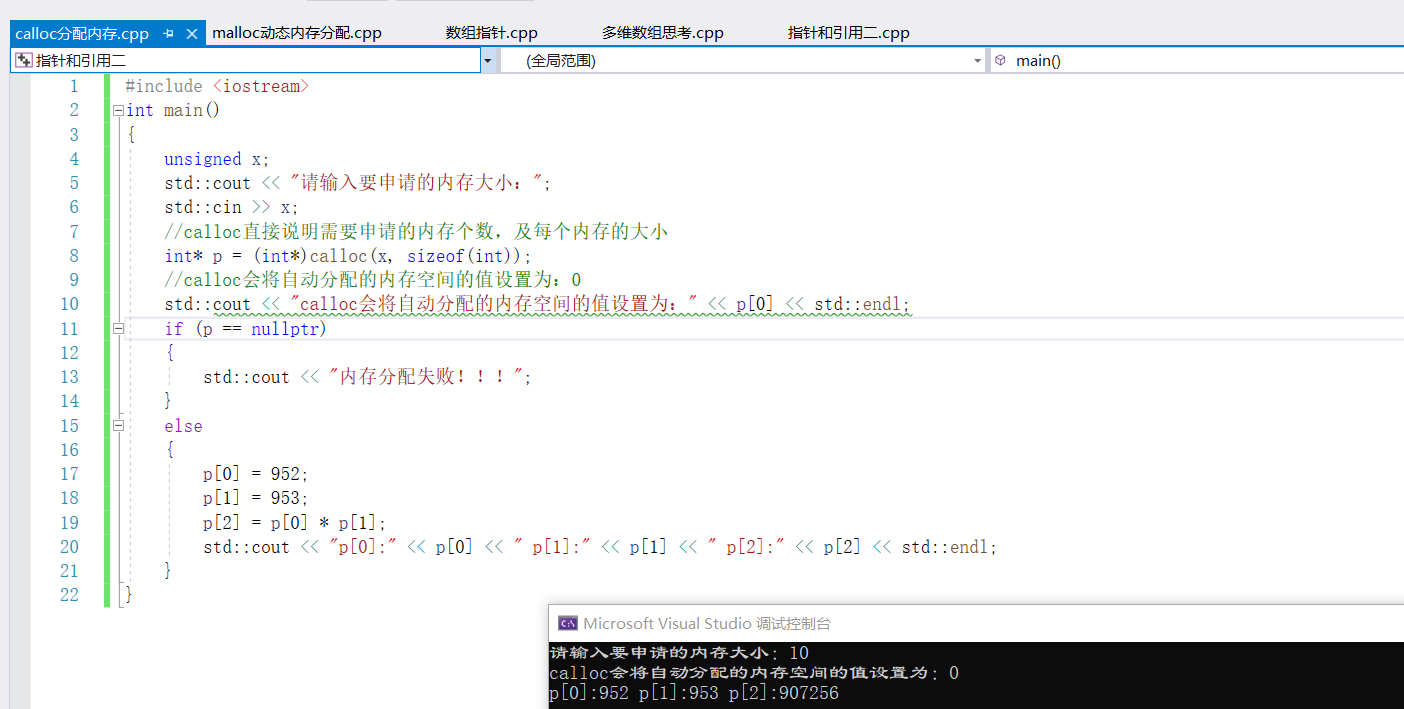3）C語言中的動態記憶體分配realloc

``````//動態記憶體分配realloc語法
void* realloc(void* _Blocak,size_t _szie); //realloc將為用戶重新分配記憶體，_Block是用戶已經分配好的記憶體，Size是要求重新分配的大小，函數返回重新分配後的記憶體

//示例
int* pa=(int*)malloc(4);
pa=(int*)realloc(pa,8);  //pa是重新分配後的記憶體地址，8是重新分配後的大小。若分配失敗，pa=0

``````
``````#include <iostream>
int main()
{
unsigned x;
std::cout << "請輸入要分配的記憶體空間大小:";
std::cin >> x;
int* p = (int*)malloc(x * sizeof(int));
if (p == nullptr)
{
std::cout << "分配記憶體失敗";
}
else
{
p = 123;
p = 456;
p = p * p;
std::cout << "p:" << p << " p:" << p << " p:" << p << std::endl;
}
std::cout << "請輸入要重新分配的記憶體空間大小：";
std::cin >> x;
p = (int*)realloc(p, x);  //註：重新分配記憶體空間後，原先的值還在。
std::cout << "p:" << p << " p:" << p << " p:" << p << std::endl;
free(p); p = 0;    //釋放記憶體空間並同時清0，
}
``````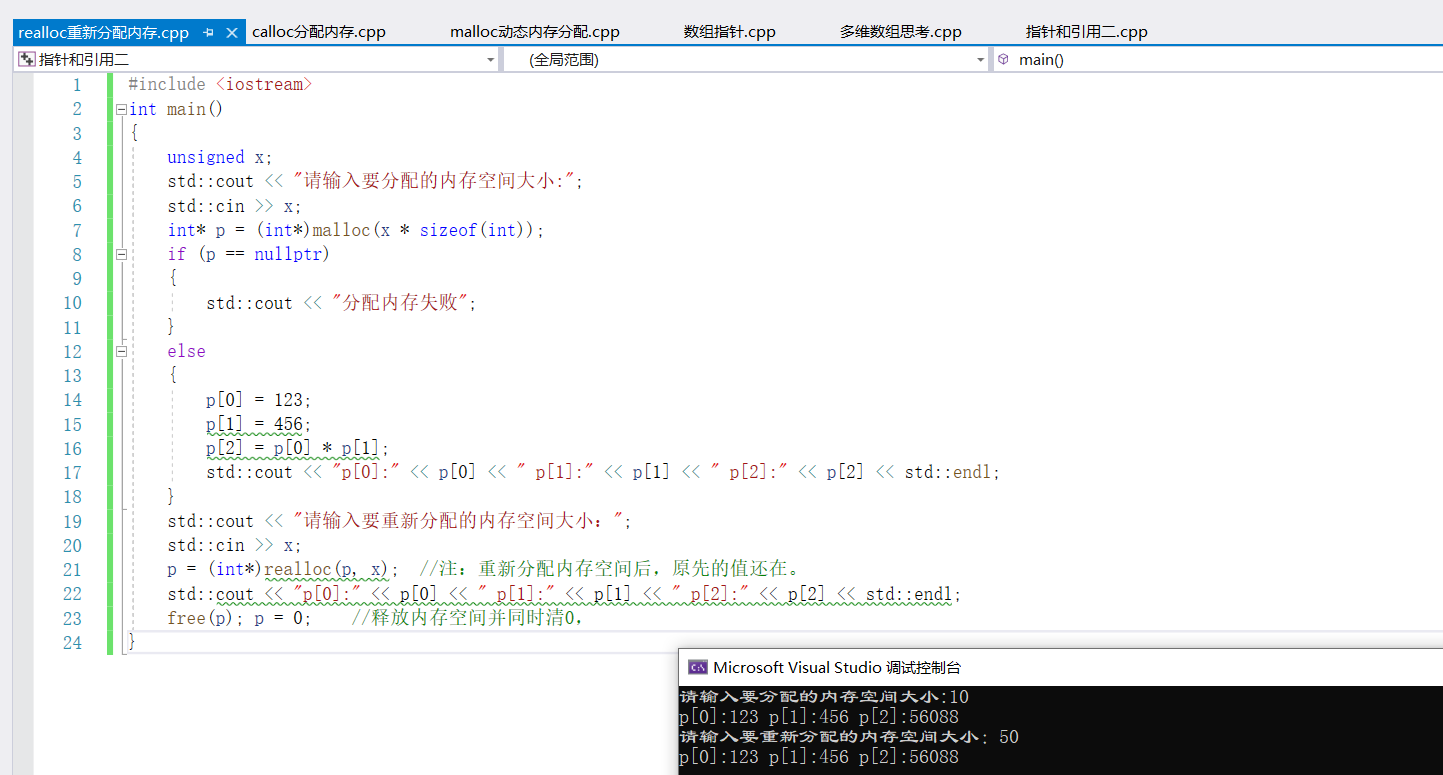4）C語言中的動態記憶體分配free

``````//釋放記憶體空間free語法
void free(void* _Blocak);

//示例
int* pa=(int*)malloc(4);
free(pa);         //pa表示所占用的記憶體被釋放，釋放記憶體不可能失敗
pa=0;             //釋放以後要將指針清零，防止出現懸掛指針
``````

5)C++的動態記憶體分配

C++記憶體分配是基於C語言的記憶體分配實現的

``````//C++的動態記憶體分配語法

//示例
int* pa = new int;
int* pa = new int;          //分配一段能夠存放5個int變數類型的記憶體空間

``````
``````//C++的動態記憶體分配語法
#include <iostream>
int main()
{
unsigned x;
std::cout << "請輸入要分配的記憶體空間大小:";
std::cin >> x;
int* pa = new int[x];   //分配存放x個int類型大小的數據
if (pa == nullptr)
{
std::cout << "分配記憶體失敗";
}
else
{
pa = 123;
pa = 456;
pa = 789;
std::cout << "pa:" << pa << std::endl;
std::cout << "pa:" << pa << std::endl;
std::cout << "pa:" << pa << std::endl;
}

}
``````
``````//反彙編
if (void* const block = malloc(size))
006227F4  mov         eax,dword ptr [size]
006227F7  push        eax
006227F8  call        _malloc (0621069h)
00622800  mov         dword ptr [ebp-4],eax
00622803  cmp         dword ptr [ebp-4],0
00622807  je          operator new+1Eh (062280Eh)

註：通過查看反彙編，可知new底層是通過malloc實現的
``````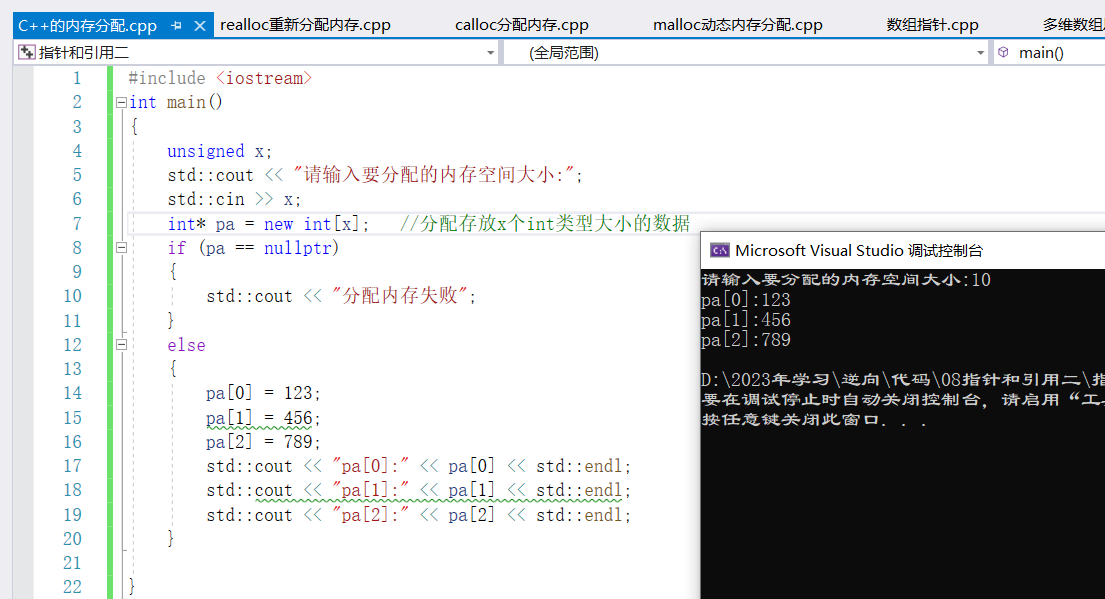6）C++記憶體釋放free

``````//C++記憶體釋放free語法
delete 指針;      //釋放用new分配的記憶體
delete[] 指針;    //釋放用new 數據類型[]分配的記憶體

``````
``````#include <iostream>
int main()
{
unsigned x;
std::cout << "請輸入要分配的記憶體空間:";
std::cin >> x;
int* p = new int[x];
int* mp = new int;
std::cout << p << std::endl;;
std::cout << p<<std::endl;

delete mp;     //釋放記憶體，如果只分配了一個，不需要加[]
delete[] p;   //釋放記憶體，如果分配了多個記憶體空間，需要加[],
}
``````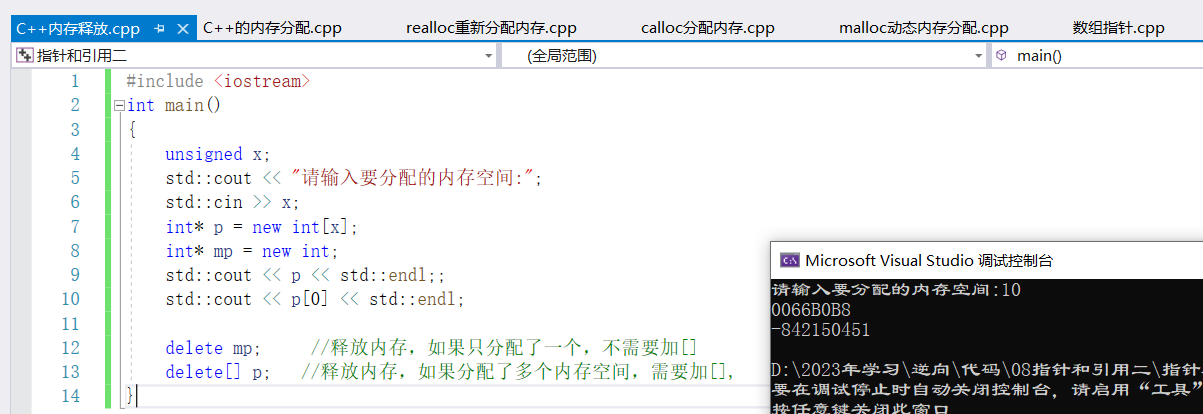##### 3、動態記憶體分配的風險

1）懸掛指針問題（野指針）

``````//通過malloc申請的記憶體，釋放以後，繼續使用不會報錯
#include <iostream>
int main()
{
int* p = (int*)malloc(10 * sizeof(int));
int* pold = p;
p = 255;
free(p);
p = 500;    //雖然p已經釋放了，但是還是去用，
//free(pold); //重覆釋放會出錯
std::cout << p << std::endl;;    //-572665370
}
``````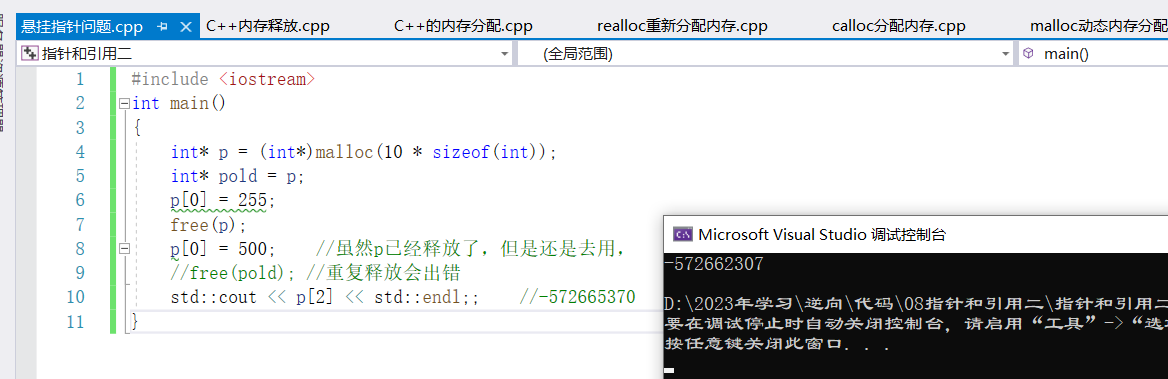``````//通過new申請的記憶體，delete釋放以後，繼續使用會報錯
#include <iostream>
int main()
{
int* p = new int;
p = 255;
delete[] p;
p = 500;    //雖然p已經釋放了，但是還是去用，
//deblete[] p; //重覆釋放會出錯
std::cout << p << std::endl;;
}
``````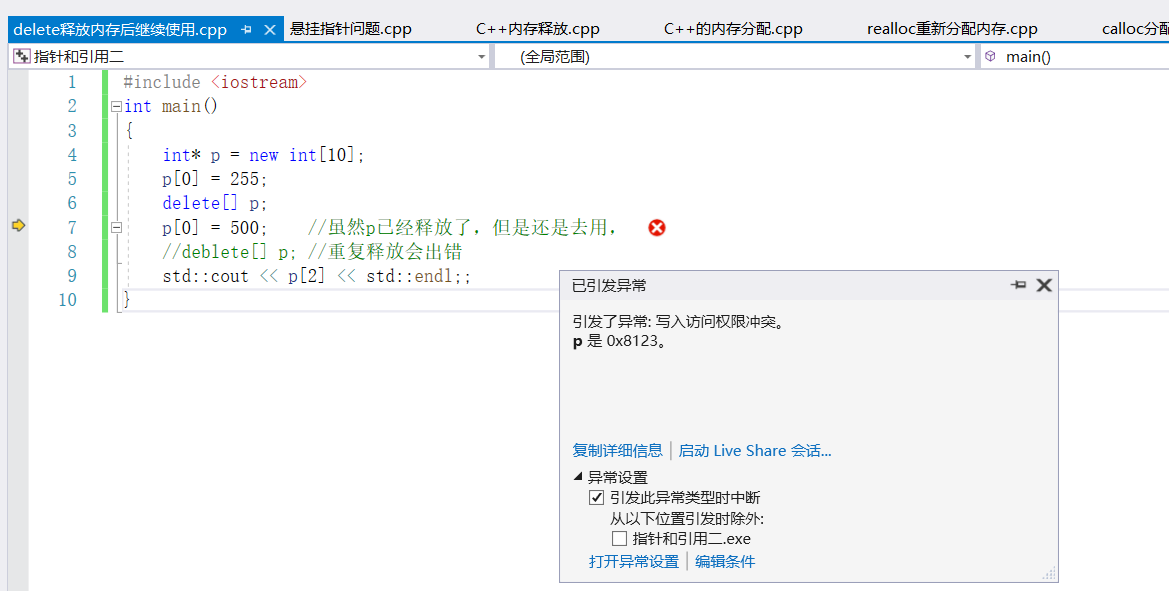``````//將new申請的記憶體，再賦值給其他變數，釋放以後，繼續使用不報錯
#include <iostream>
int main()
{
int* p = new int;
int* pa = p;
p = 255;
delete[] p;
pa = 500;    //雖然p已經釋放了，但是還是去用不會報錯，但是輸出會報錯
//deblete[] p; //重覆釋放會出錯
}
``````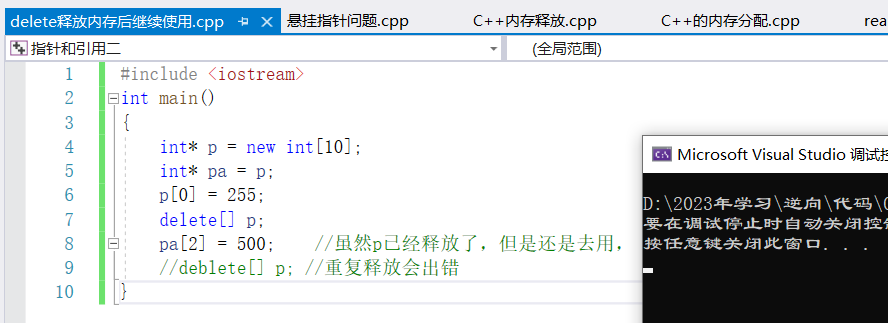``````//指針重覆釋放一定會出錯
#include <iostream>
int main()
{
int* p = new int;
int* pa = p;
delete[] p;
//delete[] pa; //指針重覆釋放一定會出錯
}
``````

2）記憶體碎片問題

​ 頻繁的申請和釋放小塊記憶體會造成記憶體碎片，雖然原則上還有記憶體可以使用，但是實際上由於剩餘記憶體碎片化的存在，使得我們無法分配新的記憶體，當然，現在也不必太擔心這樣的情況，因為new和delete背後的演算法會儘力規避這個分線，並且現在我們的虛擬記憶體也足夠大，但是如果是嵌入式開發或者要求較高的開發，就要註意這個問題，我們也可以自己來控制我們的記憶體分配，但這是比較高階的操作。

3）記憶體分配的思考

​ 原則上來說malloc分配的記憶體可以用delete釋放（new的底層是malloc），new分配的記憶體可以使用free釋放，但是實際使用時不允許兩者混用，malloc分配的記憶體一定要用free釋放、new分配的記憶體一定使用delete釋放。

4）複製記憶體memcpy

``````//複製記憶體memcpy語法
void* memcpy(void* _Dst,const void* _Src,size_t _size);  //memcpy可以將_Src區域的記憶體複製到_Dst區域，複製的長度為_Size,賦值的單位為位元組

//示例
int a{1001,1002,1003,1004,1005};
int *p = new int;
memcpy(p,a,5*sizeof(int));
``````
``````//複製記憶體，將數組中的數據，複製到指針數組中
#include <iostream>

int main()
{
int a{ 1001,1002,1003,1004,1005 };
int* p = new int;
/*
法一：
for (int i = 0; i < 5; i++)
{
p[i] = a[i];
}
*/
法二
memcpy(p, a, 5 * sizeof(int));      //單位為位元組
for (int j = 0; j < 5; j++)
{
std::cout << p[j]<<std::endl;
}
}
``````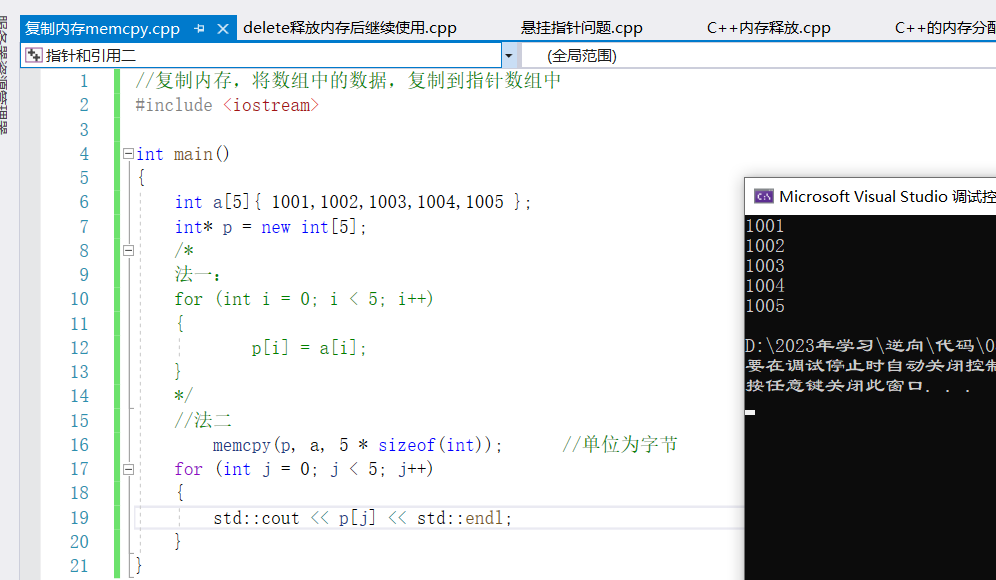4）設置記憶體memset

``````//設置記憶體memset語法
void* memset(void* _Dst,int val,size_t _Size);  //memset可以將指定記憶體區域每一個位元組的值都設置為val,_Size表示要設置的長度（單位：位元組）

//示例
int* p=new int;
memset(p,0,100*sizeof(int));

``````
``````#include <iostream>

int main()
{
int* p = new int;
memset(p, 0, 100 * sizeof(int));      //設置記憶體，[地址，設置的值，設置的大小],不管設置的大小為多少，只能一個位元組一個位元組初始化
//memset(p, 0xff, 100 * sizeof(int));     //初始化記憶體為ffffff
std::cout << p << std::endl;;
}
``````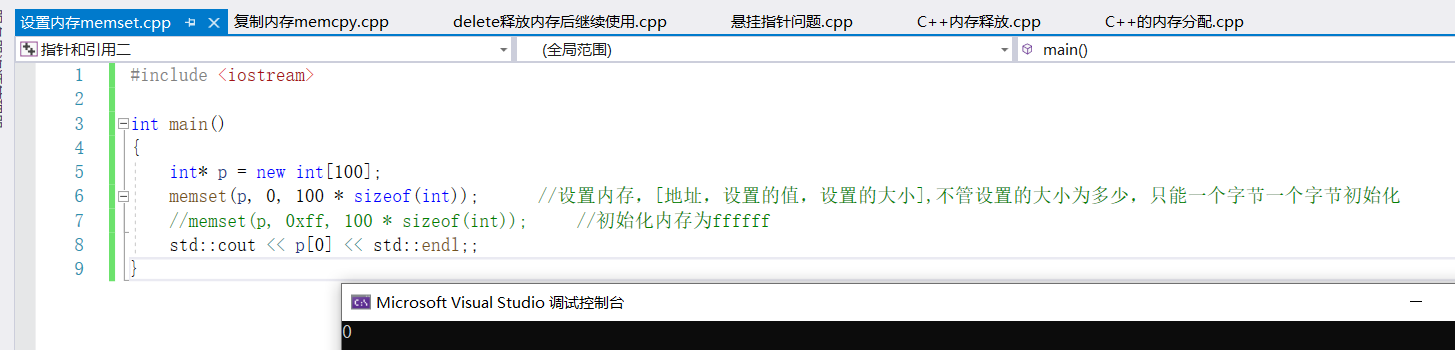##### 4、引用類型

``````//引用語法

//示例
int a{500};
int& la{a};    //以後對la的操作就相當於對a的操作，修改la就相當於修改了a

``````
``````//引用使用
#include <iostream>
int main()
{
int a{ 5250 };
//引用誰就等於誰，即將a起了另外一個名字,將a的值複製給la，包括地址。一個變數可以有多個引用
int& la{ a };
int& la1{ a };
int& la2{ a };
int& la3{ a };

//常量的引用
const int b{ 3000 };
const int& lb{ b };
std::cout << lb << std::endl;  //3000

std::cout << &a << std::endl;     //00cBEFF8
a++;
std::cout << la << std::endl;   //a++就相當於la++
std::cout << &la1 << std::endl;   //00cBEFF8
std::cout << &la2 << std::endl;
std::cout << &la3 << std::endl;
}
``````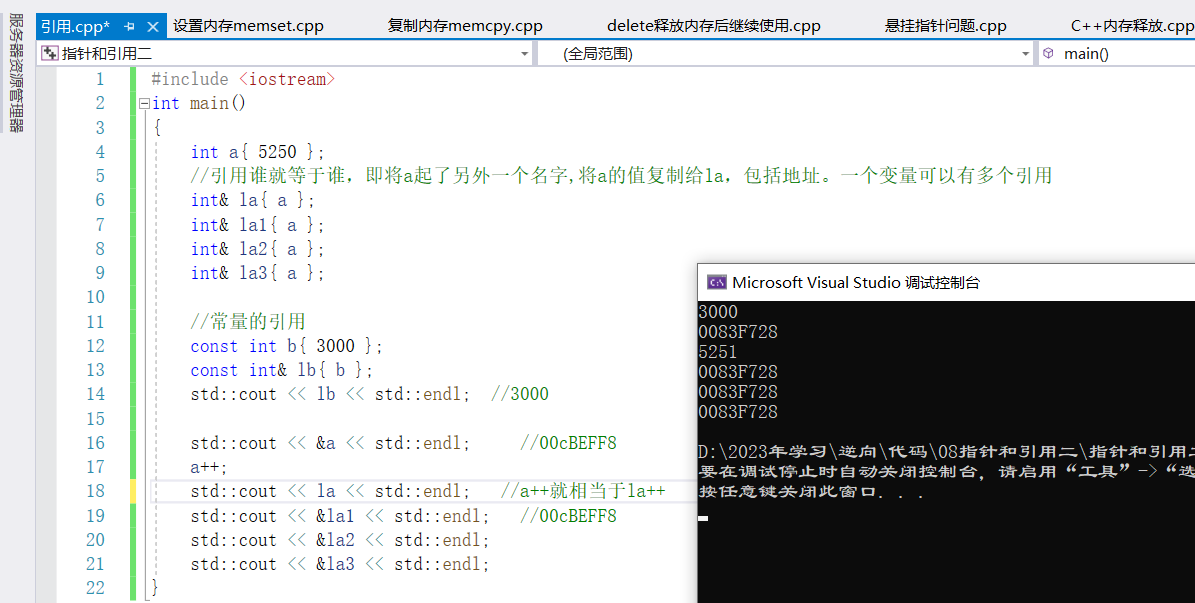##### 5、指針練習

​ 暗網殺手排名系統：暗網是一個不為人知的網路世界，在暗網中有10位臭名昭著的殺手，如果按照臭名值來排名，分別是：

​ 正序排列：105,98,73,58,32,31,25,22,3,1

​ 倒序排列：1,3,2,25,31,32,58,73,98,105

​ 現在我們要給一位新來的殺手插入到這個排名系統中去要求設計一個演算法，要求用戶輸入一個值代表新殺手的臭名值，然後不管是正序排列，還是倒序排列，這個演算法都能夠正確的將新殺手的臭名值正確的排序到現有排序中去！並且列印出來比如：用戶輸入97則輸出：

105,98,97,73,58,32,31,25,22,3,1或者是1,3,22,25,31,32,58,73,98,105

!

``````#include <iostream>

int main()
{
//int a{ 105,98,73,58,32,31,25,22,3,1 };
int a{ 1,3,22,25,31,32,58,73,98,105 };
int acount = sizeof(a) / sizeof(int);       //計算數組a的元素個數
int* anew = new int[acount + 1];         //新的數組

int killer;
std::cout << "請輸入新殺手的臭名值：";
std::cin>> killer;

int getIndex{ acount };       //插入的索引位置

if (a > a)
{
for (int i = 1; i < acount; i++)
{
if (killer > a[i])
{
getIndex = i;
break;
}
}
}
if (a < a)
{
for (int i = 1; i < acount; i++)
{
if (killer < a[i])
{
getIndex = i;
break;
}
}
}
memcpy(anew, a, getIndex * sizeof(int));  //拷貝插入位置之前的數據
memcpy(anew + getIndex + 1, a + getIndex, (acount - getIndex)*sizeof(int));  //拷貝插入位置之後的數據
anew[getIndex] = killer;
for (int i = 0; i <= acount; i++)
{
std::cout << anew[i] << std::endl;
}
//std::cout << getIndex << std::endl;
}
``````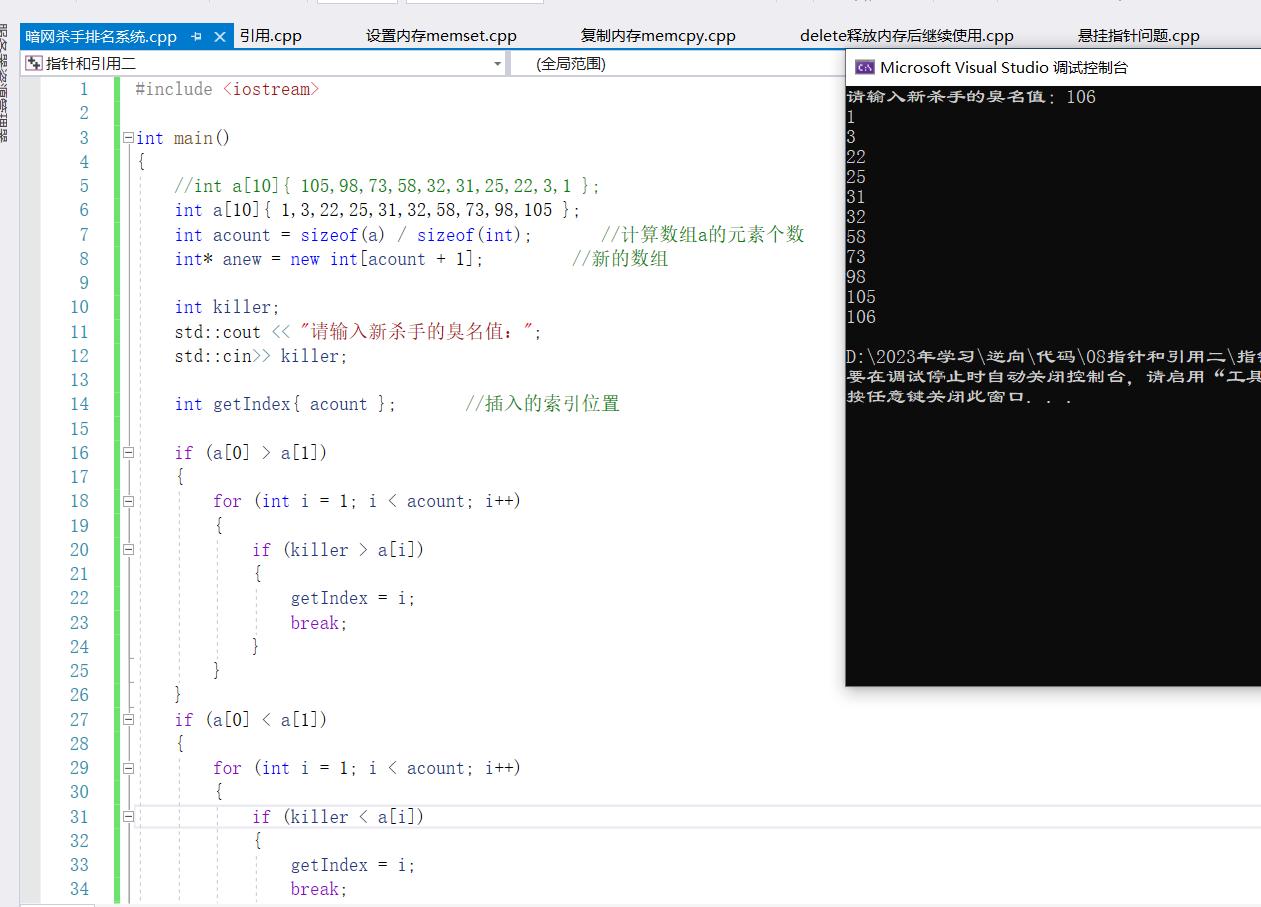``````//上述代碼優化(實現效果一致)
#include <iostream>

int main()
{
//int a{ 105,98,73,58,32,31,25,22,3,1 };
int a{ 1,3,22,25,31,32,58,73,98,105 };
int acount = sizeof(a) / sizeof(int);       //計算數組a的元素個數
int* anew = new int[acount + 1];         //新的數組

int killer;
std::cout << "請輸入新殺手的臭名值：";
std::cin >> killer;

int getIndex{ acount };       //插入的索引位置

bool bcase = a > a;           //代碼優化

for (int i = 0; i < acount; i++)
{
if (bcase ^ (killer < a[i]))
{
getIndex = i;
break;
}

}
memcpy(anew, a, getIndex * sizeof(int));  //拷貝插入位置之前的數據
memcpy(anew + getIndex + 1, a + getIndex, (acount - getIndex) * sizeof(int));  //拷貝插入位置之後的數據
anew[getIndex] = killer;
for (int i = 0; i <= acount; i++)
{
std::cout << anew[i] << std::endl;
}
//std::cout << getIndex << std::endl;
}

/*
for(int i=0;i<acount;i++)
{
if(a>a)
{
if(x>a[i])
{
getIndex = i;
break;
}
}
else
{
if(x<a[i])
{
getIndex=i;
break;
}
}
}
*/
``````
##### 12、理解數組和指針

1）數組和指針

``````int a;
int c = sizeof(a);
006E17D8 mov dword ptr [c],14h
``````

2）引用的本質

3）引用的分析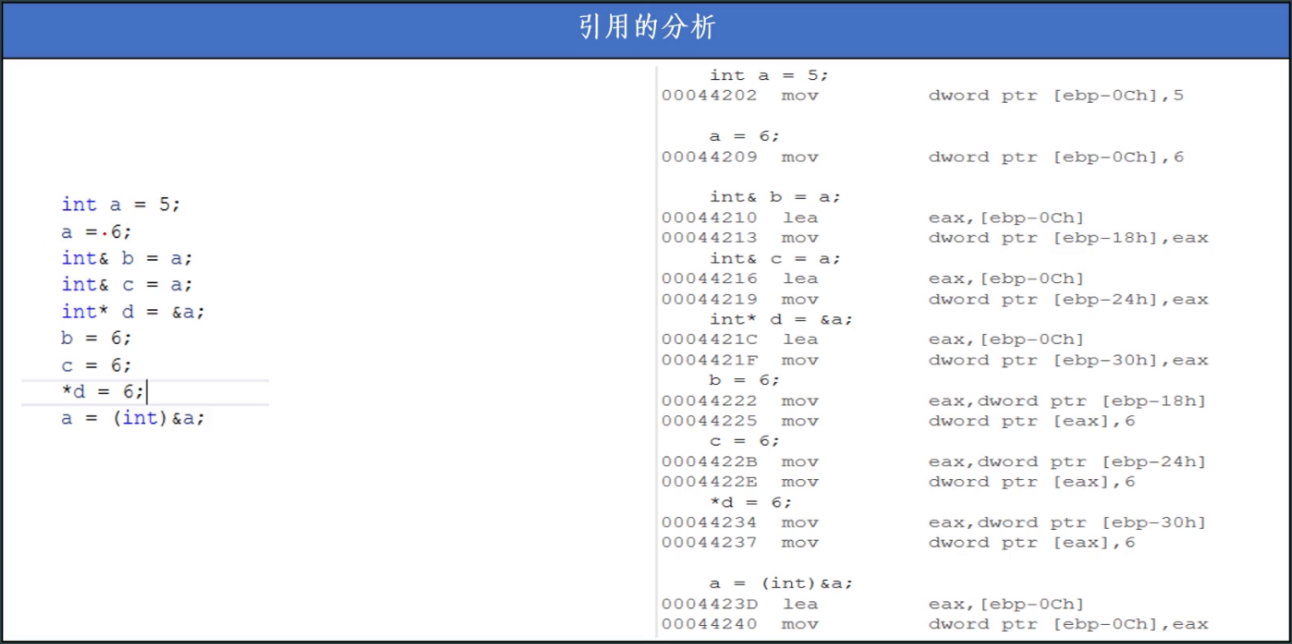4）堆和棧

​ 堆的本質其實就是空閑記憶體，C++中把堆稱為自由儲存區，只要是你的程式載入後，沒有占用的空閑記憶體，都是自由存儲區，我們用new或者malloc申請一塊新的記憶體區域，都是由操作系統從堆上操作。

​ 棧是程式在編譯時就已經確定了大小的一段記憶體區域，主要是用於臨時變數的存儲，棧的效率要高於堆，但是容量有限。

##### 13、技能系統（一）

1）技能系統

​ 需求：本次重新設計麟江湖的技能系統，技能是在游戲設計之處已經設計好的，游戲設計了十一種技能，屬性如下：

2）項目設計

​ 設計需求：每個角色隨著等級不同，最少擁有一個技能，即普通攻擊；最多擁有5個技能，技能擁有等級，技能等級每提升1級，角色的最終傷害提升15%，設計角色的屬性面板，能夠顯示角色的屬性和技能，角色屬性根據需求自己發揮。

``````#include <iostream>

struct Skill                //定義技能結構體
{
char Name; //技能名稱
int Mpp; //內力消耗
int Spp; //怒氣
int Act; //攻擊力
int ActB; //翻倍攻擊
int CoolDown; //冷卻時間
};

struct USkill        //定義用戶當前的技能
{
Skill* skill{};
int lv;
int cooldown;
bool buse{};
};

typedef struct Role
{
char Name;
int HP;
int MaxHp;
int MP;
int MaxMp;
int Sp;     //怒氣值
int MaxSp;
int Act;    //普通攻擊力
USkill skills;   //每個角色最多5技能
}*PROLE;

int main()
{
Skill AllSkills
{
{"普通攻擊",0,0,10,1,0},
{"大力金剛指",10,0,50,1,1},
{"雲龍三觀",10,0,60,1,1},
{"一陽指",30,0,0,2,3},
{"迎風破浪",30,0,300,0,3},
{"八卦掌",50,0,0,5,4},
{"六合八荒",50,0,500,0,4},
{"仙人指路",100,0,0,10,6},
{"橫掃千軍",100,0,50,2,6},
{"氣吞山河",0,100,500,5,0},
{"秋分刀法",0,100,200,0,0}
};

PROLE User = new Role
{
"郝英俊",
1000,1000,1000,1000,0,100,100,
{
{&AllSkills,0,0,true},
{&AllSkills,0,0,true},
{&AllSkills,0,0,false},
{&AllSkills,0,0,false},
{&AllSkills,0,0,true}
}
};

PROLE Monster = new Role
{
"凹凸曼",
1000,1000,1000,1000,0,100,100,
{
{&AllSkills,0,0,true},
{&AllSkills,0,0,true},
{&AllSkills,0,0,true},
{&AllSkills,0,0,true},
{&AllSkills,0,0,true}
}
};

std::cout << "角色姓名:"<<User->Name << std::endl;
std::cout << "生命:" << User->HP << "/" << User->MaxHp << std::endl;
std::cout << "內力:" << User->MP << "/" << User->MaxMp << std::endl;
std::cout << "怒氣:" << User->Sp << "/" << User->MaxSp << std::endl;
std::cout << "基本攻擊:" << User->Act << std::endl;

for (auto skill : User->skills)
{
if (skill.buse)
std::cout
<<"技能名稱 ["<<skill.skill->Name<<"]"
<< "消耗MP " << skill.skill->Mpp
<< "消耗SP " << skill.skill->Spp
<< "附加攻擊 " << skill.skill->Act
<< "翻倍攻擊 " << skill.skill->ActB
<< "冷卻 " << skill.skill->CoolDown
<< "技能等級 " << skill.lv
<< std::endl;

}
}
``````• 這裡給大家分享我在網上總結出來的一些知識，希望對大家有所幫助 效果 金幣從初始位置散開後逐個飛向指定位置，這是游戲中很常用的一個動畫，效果如下： 思路 這個效果中，分成兩個階段： 一定數量的金幣從一個起點散開 這些金幣逐一飛向終點 計算金幣的初始散開位置 生成圓周上的等分點 金幣散開的位置看似隨機， ...
• 古詩文起名 大家好，我是 Java陳序員，我們常常會為了給孩子取名而煩惱，取名不僅要好聽而且要規避大眾化。其實，我們中華文化博大精深，可以借鑒先輩文人們留下的經典詩詞中的文字來起名。今天，給大家介紹一個古詩文起名的工具。 這個工具支持從《詩經》、《楚辭》、《唐詩》、《宋詞》、《樂府詩集》、《古詩三百 ...
• SubScribe即發佈訂閱模式，在工作中有著廣泛的應用，比如跨組件通信，微前端系統中跨子應用通信等等。 以下是一個簡易的實現： 訂閱 初始化時可限制類型 發佈 限制類型是為了讓訂閱者和發佈者知道預製了哪些類型，避免使用了一些對方不知道的類型。 type Subscriber<T> = (param ...
• 使用集團的統一埋點採集能力和埋點平臺，完成達達7條業務線共43個站點應用的埋點遷移，降低自研採集工具和平臺的研發投入和機器成本，打通數據鏈路，創造更多的數據分析價值 ...
• 大家好，我是Java陳序員。 我們在日常開發中，會有很多的應用環境，開發環境、測試環境、回歸環境、生產環境等等。 這些環境，需要部署在一臺台的伺服器上，有的可能是物理機，有的可能是雲伺服器。 那麼，這麼多主機我們要怎麼運維整理呢？ 今天，給大家介紹一個輕量級的自動化運維平臺。 項目介紹 Spug—— ...
• Vue3中響應數據核心是 reactive ， reactive 中的實現是由 proxy 加 effect 組合，我們先來看一下 reactive 方法的定義 ...
• 背景 近期查看公司項目的請求日誌，發現有一段來自俄羅斯首都莫斯科（根據IP是這樣，沒精力溯源）的異常請求，看傳參就能猜到是EXP攻擊，不是瞎掃描瞎傳參的那種。日誌如下（已做部分修改）： [2023-11-17 23:54:34] local.INFO: url : http://xxx/_ignit ...